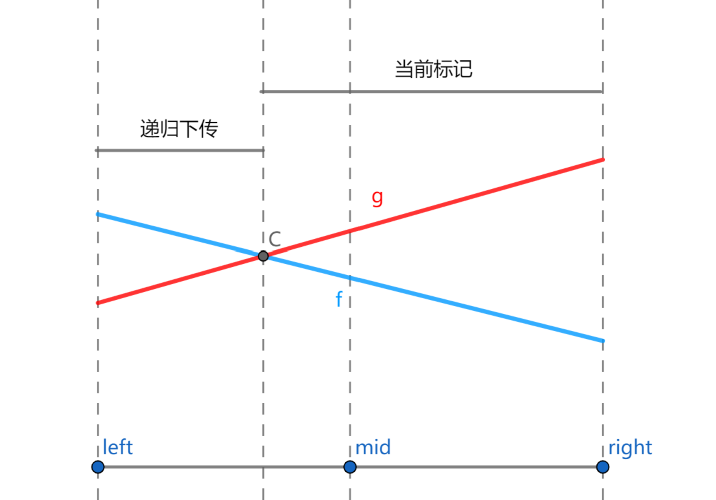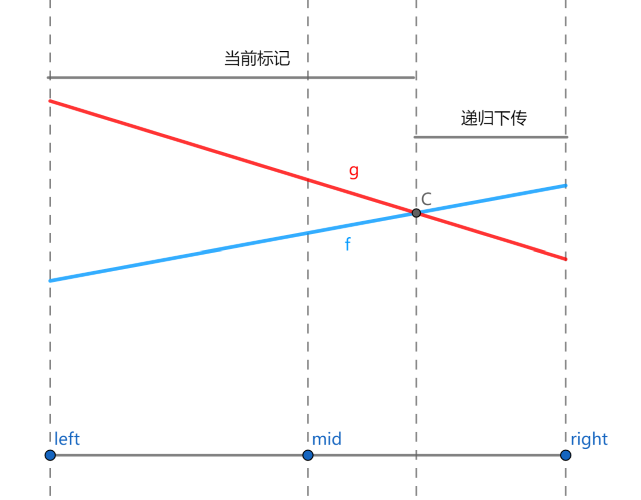# 李超线段树笔记

## 线段树之标记永久化

1. 比如我们先设置后加，并且令设置的区间比加的区间大，因此加的 tag 在下方，设置的 tag 在上方。
2. 根据前面的方法，我们会从下往上取 tag，也就是先加法，再设置。
3. 这时我们发现，由于上层是一个设置操作，下面的所有答案最终都变成了设置的那个数字，下层操作就失效了。显然有问题。

  1 2 3 4 5 6 7 8 9 10 11 12 13 14 15 16 17 18 19  int up(int p) { tree[p].mx = max(tree[p<<1].mx, tree[p<<1|1].mx)+tree[p].tag; } int query(int p, int l, int r, int x, int y) { if (l >= x && r <= y) return tree[p].mx; int mid = (l + r) / 2, ans = -INF; if (x <= mid) ans = max(ans, query(p << 1, l, mid, x, y)); if (y > mid) ans = max(ans, query(p << 1 | 1, mid + 1, r, x, y)); return ans+tree[p].tag; } void update(int cur, int l, int r, int x, int y, int c) { if (l >= x && r <= y) { tree[cur].tag += c; tree[cur].mx += c; return; } int mid = (l + r) / 2; if (x <= mid) update(cur << 1, l, mid, x, y, c); if (y > mid) update(cur << 1 | 1, mid + 1, r, x, y, c); up(cur); } 

## 李超线段树

1. 在平面上加入一条线段。记第 $i$ 条被插入的线段的标号为 $i$，该线段的两个端点分别为 $(x_0,y_0)$，$(x_1,y_1)$。
2. 给定一个数 $k$，询问与直线 $x = k$ 相交的线段中，交点纵坐标最大的线段的编号（若有多条线段与查询直线的交点纵坐标都是最大的，则输出编号最小的线段）。特别地，若不存在线段与给定直线相交，输出 $0$。

• 加入一个一次函数，定义域为 $[l,r]$；
• 给定 $k$，求定义域包含 $k$ 的所有一次函数中，在 $x=k$ 处取值最大的那个，如果有多个函数取值相同，选编号最小的。1. 如果新线段 $f$ 更优，则将 $f$ 和 $g$ 交换。转化成了第 2 种情况。
2. 对于中点处 $f$ 不如 $g$ 优的情况：
• 若在左端点处 $f$ 更优，那么 $f$ 和 $g$ 必然在左半区间中产生了交点，$f$ 只有在左区间才可能优于 $g$，递归到左儿子中进行下传；
• 若在右端点处 $f$ 更优，那么 $f$ 和 $g$ 必然在右半区间中产生了交点，$f$ 只有在右区间才可能优于 $g$，递归到右儿子中进行下传；
• 若在左、右端点处 $g$ 都更优，那么 $f$ 不可能成为答案，不需要继续下传。

RECORD

  1 2 3 4 5 6 7 8 9 10 11 12 13 14 15 16 17 18 19 20 21 22 23 24 25 26 27 28 29 30 31 32 33 34 35 36 37 38 39 40 41 42 43 44 45 46 47 48 49 50 51 52 53 54 55 56 57 58 59 60 61 62 63 64 65 66 67 68 69 70 71 72 73 74 75 76 77 78 79 80 81 82 83 84 85 86 87 88 89 90 91 92 93 94 95 96 97 98 99 100 101 102 103 104 105 106 107 108 109 110 111 112  #include using namespace std; const int N = 1000005; const int MOD1 = 39989; const int MOD2 = 1000000000; const double EPS = 1e-12; struct line { double k, b; int l, r; int id; } tree[N << 2]; int n; double calc(line a, int x) { // 计算纵坐标 return x * a.k + a.b; } // 注意 cmp 写法。不要写成 a-b < EPS。 int cmp(double a, double b) { if (a - b > EPS) return 1; if (b - a > EPS) return -1; return 0; } void build(int rt, int l, int r) { tree[rt] = {0, 0, 1, 40000, 0}; if (l == r) return; int m = (l + r) >> 1; build(rt << 1, l, m); build(rt << 1 | 1, m + 1, r); } void modify(int root, int l, int r, line k) { if (l >= k.l && r <= k.r) { // 对线段完全覆盖到的区间进行修改 // g: tree[root]; f: k. int m = (l + r) >> 1; if (cmp(calc(tree[root], m), calc(k, m)) == -1) swap(tree[root], k); int cl = cmp(calc(k, l), calc(tree[root], l)), cr = cmp(calc(k, r), calc(tree[root], r)); // 3. 若在左右端点处 g 都更优，那么 f 不可能成为答案，不需要继续下传。 if (cl == -1 && cr == -1) return; // 1. & 2. 注意题目要求下标尽量小，下标小的线段即使不更优，如果值相等，也可以下传。 if (cl == 1 || (cl == 0 && k.id < tree[root].id)) modify(root << 1, l, m, k); if (cr == 1 || (cr == 0 && k.id < tree[root].id)) modify(root << 1 | 1, m + 1, r, k); } else { int m = (l + r) >> 1; if (k.l <= m) modify(root << 1, l, m, k); if (k.r > m) modify(root << 1 | 1, m + 1, r, k); } } pair pmax(pair x, pair y) { // 注意题目要求下标尽量小 if (cmp(x.first, y.first) == -1) return y; else if (cmp(x.first, y.first) == 1) return x; else return x.second < y.second ? x : y; } pair query(int root, int l, int r, int x) { if (l == r) return {calc(tree[root], x), tree[root].id}; int m = (l + r) >> 1; pair ans = {calc(tree[root], x), tree[root].id}; // 注意标记永久化，取 max 比较 if (x <= m) ans = pmax(ans, query(root << 1, l, m, x)); else ans = pmax(ans, query(root << 1 | 1, m + 1, r, x)); return ans; } int main() { ios::sync_with_stdio(false); cin >> n; build(1, 1, 40000); int lastans = 0; int segi = 0; while (n--) { int op; cin >> op; if (op == 0) { int k; cin >> k; k = (k + lastans - 1 + MOD1) % MOD1 + 1; lastans = query(1, 1, 40000, k).second; cout << lastans << endl; } else { int a, b, x, y; cin >> a >> b >> x >> y; a = (a + lastans - 1 + MOD1) % MOD1 + 1; b = (b + lastans - 1 + MOD2) % MOD2 + 1; x = (x + lastans - 1 + MOD1) % MOD1 + 1; y = (y + lastans - 1 + MOD2) % MOD2 + 1; if (a > x) { swap(a, x); swap(b, y); } line t; if (a == x) { // 垂直于 y 的函数的特殊处理 t.k = 0; t.b = max(b, y); } else { t.k = (double)(y - b) / (x - a); t.b = b - t.k * a; } t.l = a; t.r = x; t.id = ++segi; modify(1, 1, 40000, t); } } return 0; }